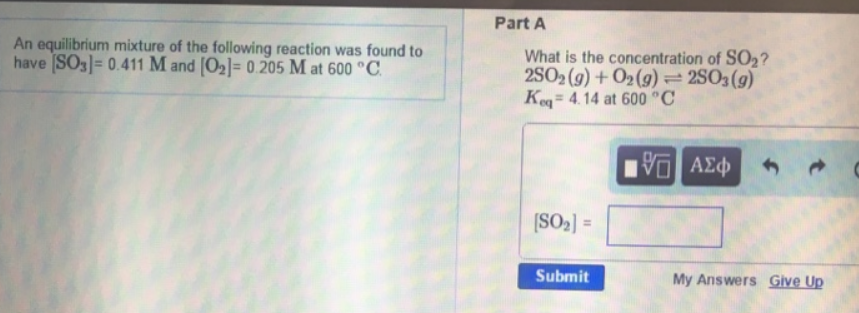# Problem: An equilibrium mixture of the following reaction was found to have [SO3] = 0.411 M and [O2] = 0.205 M at 600°C What is the concentration of SO2?2 SO2(g) + O2(g) ⇌ 2 SO3(g)Keq = 4.14 at 600 °C

🤓 Based on our data, we think this question is relevant for Professor Gillespie's class at FIU.

###### FREE Expert Solution###### Problem Details

An equilibrium mixture of the following reaction was found to have [SO3] = 0.411 M and [O2] = 0.205 M at 600°C What is the concentration of SO2?

2 SO2(g) + O2(g) ⇌ 2 SO3(g)
Keq = 4.14 at 600 °C